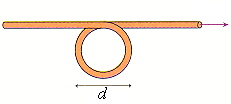# Problem: What is the strength of the magnetic field at the center of the loop in the figure? Assume that I = 6.0 A and diamter = 2.5 cm

###### FREE Expert Solution

The magnetic field at the center of the loop is given by the sum of the magnetic field due to current in the long wire and the magnetic field due to current in the other long wire.

92% (366 ratings)###### Problem Details

What is the strength of the magnetic field at the center of the loop in the figure? Assume that I = 6.0 A and diamter = 2.5 cm